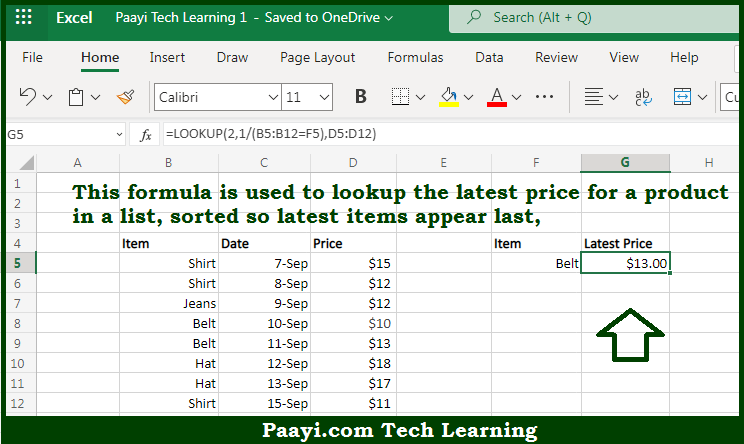# Learn How to Lookup Latest Price in Microsoft Excel

Written by | 0 Comments | 291 Views

In this article, you will learn how to evaluate things based on the LOOKUP function in Microsoft Excel using a single/combination(s) of functions. You will also know how to Lookup Latest Price and see the generic formula.

Learn How to Lookup Latest Price in Microsoft Excel

The main purpose of this formula is to lookup the latest price for a product in a list, sorted so the latest items appear last. Here we will learn how to perform lookup for the latest price in the given data range in the workbook in Microsoft Excel. That implies, with the help of a formula based on the LOOKUP function you can able to lookup the latest price for a product in a list, sorted so the latest items appear last. So, with the help of this formula, you can able to perform lookup for the latest price in the given data range in the workbook in Microsoft Excel.

General Formula to Lookup Latest Price

=LOOKUP(2,1/(item="hat"),price)

The Explanation to Lookup Latest PriceSo we know that with the help of the given formula above you can able to lookup the latest price for a product in a list, sorted so the latest items appear last. Here we will learn how to perform lookup for the latest price in the given data range in the workbook in Microsoft Excel. As we know the LOOKUP function assumes data is sorted, and always does an approximate match. If the lookup value is greater than all values in the lookup array, the default behavior is to "fall back" to the previous value. This formula exploits this behavior by creating an array that contains only 1s and errors, then deliberately looking for the value 2, which will never be found.  So now you have learned how to perform lookup for the latest price in the given data range in the workbook in Microsoft Excel.